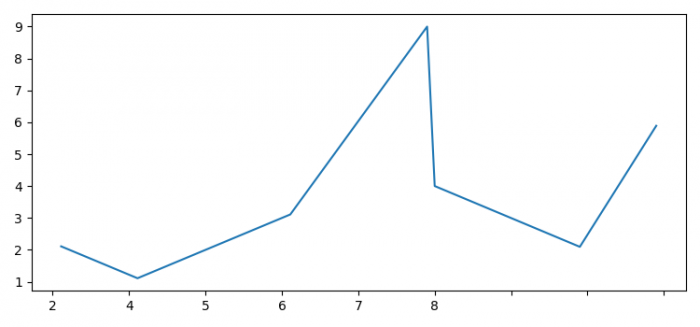# How to remove the digits after the decimal point in axis ticks in Matplotlib?

To remove the digits after the decimal point in axis ticks in Matplotlib, we can take the following steps −

• Set the figure size and adjust the padding between and around the subplots.

• Create x and y data points using numpy.

• Create a figure and a set of subplots.

• To set the xtick labels only in digits, we can use x.astype(int) method.

• To display the figure, use show() method.

## Example

import matplotlib.pyplot as plt
import numpy as np

plt.rcParams["figure.figsize"] = [7.50, 3.50]
plt.rcParams["figure.autolayout"] = True

x = np.array([1.110, 2.110, 4.110, 5.901, 6.00, 7.90, 8.90])
y = np.array([2.110, 1.110, 3.110, 9.00, 4.001, 2.095, 5.890])

fig, ax = plt.subplots()

ax.plot(x, y)

ax.set_xticklabels(x.astype(int))

plt.show()

## Output